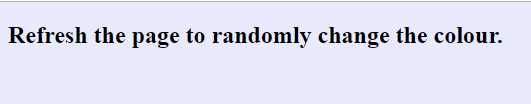## Tuesday 24 December 2019

### Webpage background colour changer

The following JavaScript code is designed to randomly change the background colour of the webpage each time it is refreshed.Screenshot of webpage colour

We start by creating an 'array' of different colours we want to use for the webpage background.
var bgColour = ["#029FD4", "#D43702", "#1EFF00", "#EAEAFF", "#FFEA00", "#AB5252", "#FFFFFF", "#DE28D8"];
Next we make use of 'Math.random()' to generate a random number greater than or equal to 0.0 and less than 1.0 and we multiply this by the length of the above array (so 8 in this instance).
var arrayPosition = Math.random() * bgColourLength;
From this we get a random number that contains multiple decimal places, so we can use 'Math.floor()' to round to the nearest whole number.
var roundNumber = Math.floor(arrayPosition);
Now we can change the webpage background colour by using the randomly generated whole number as the index position in the colour array.
document.body.style.background=bgColour[roundNumber];

Webpage background colour changer.html